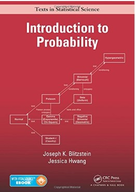Introduction To Probability - 1 Edition - Chapter 3 - Problem 32
Register Now

Join StudySoup

Get Full Access to Introduction To Probability - 1 Edition - Chapter 3 - Problem 329781466575578

# In Evans history class, 10 out of 100 key terms will be randomly selected to appear on

Introduction to Probability | 1st Edition

Problem 32

In Evans history class, 10 out of 100 key terms will be randomly selected to appear on the final exam; Evan must then choose 7 of those 10 to define. Since he knows the format of the exam in advance, Evan is trying to decide how many key terms he should study. (a) Suppose that Evan decides to study s key terms, where s is an integer between 0 and 100. Let X be the number of key terms appearing on the exam that he has studied. What is the distribution of X? Give the name and parameters, in terms of s. (b) Using R or other software, calculate the probability that Evan knows at least 7 of the 10 key terms that appear on the exam, assuming that he studies s = 75 key terms.

Accepted Solution
Step-by-Step Solution:
Step 1 of 3

Statistics in Health and Biology 8/22/16 1:46 PM Chapter 1 Section 1.1 Descriptive statistics: Consists of methods for organizing and summarizing information; it includes the construction of graphs, charts, and tables, and the calculation of various descriptive measures such as averages, measures of variation, and percentiles. Population: The collection of all individuals or items under consideration in a statistical study. Sample: The part of the population from which information is obtained. Parameter: Numerical values describing a population. Statistics: Numerical values describing a sample. Inferential Statistics: Consists of methods for drawing and measuring the reliability of conclusio

###### Chapter 3, Problem 32 is Solved

Step 2 of 3

Step 3 of 3

Unlock Textbook Solution